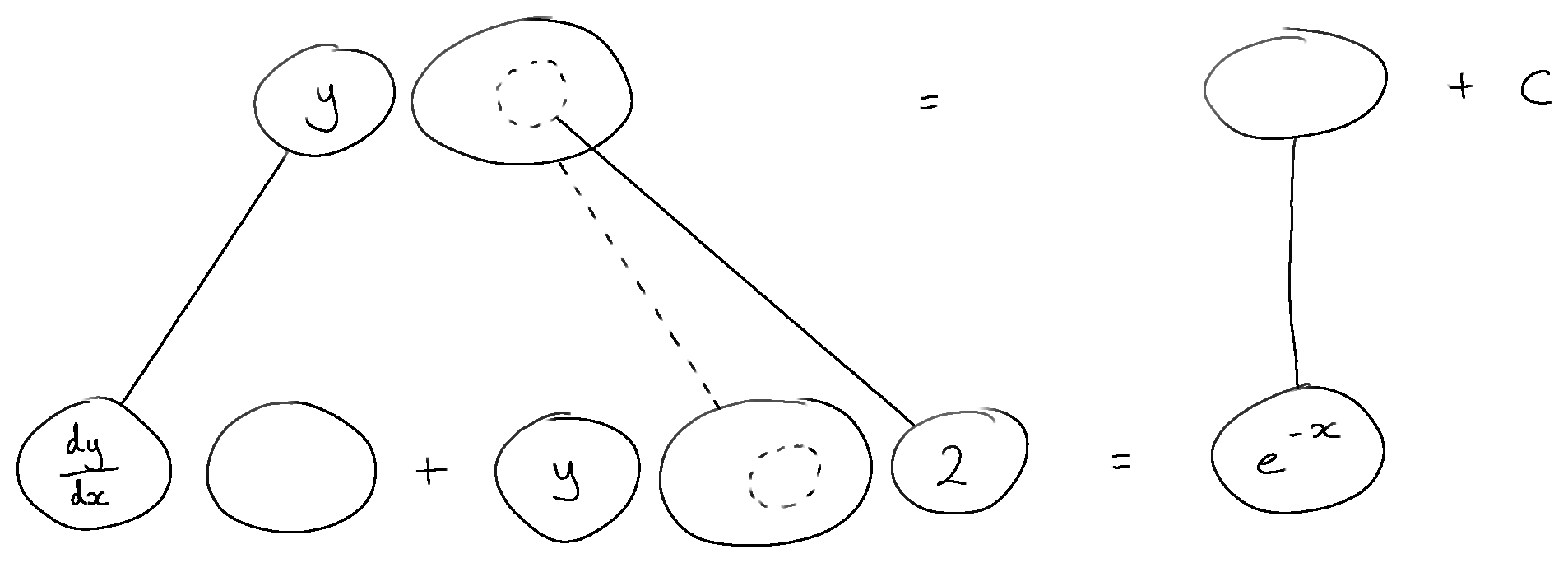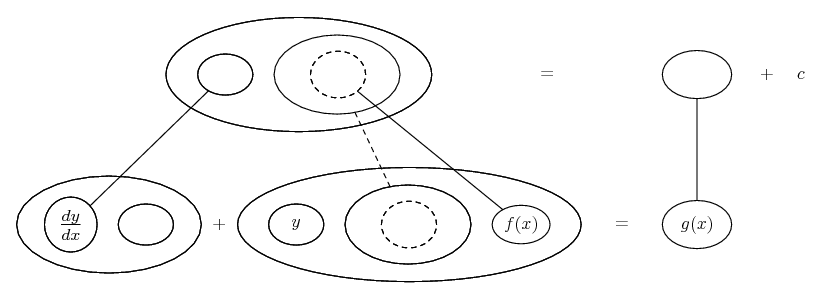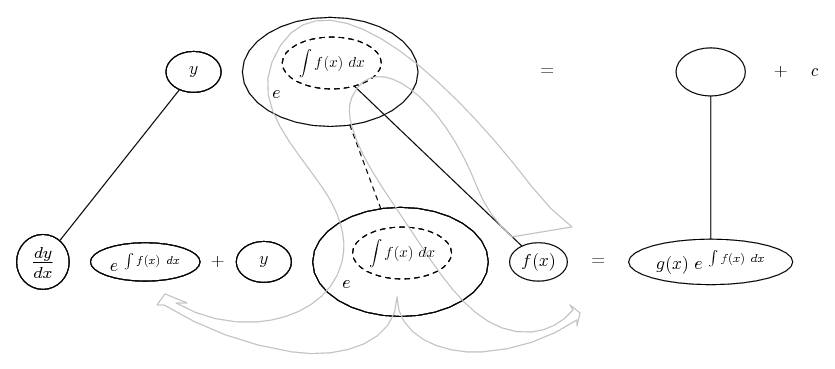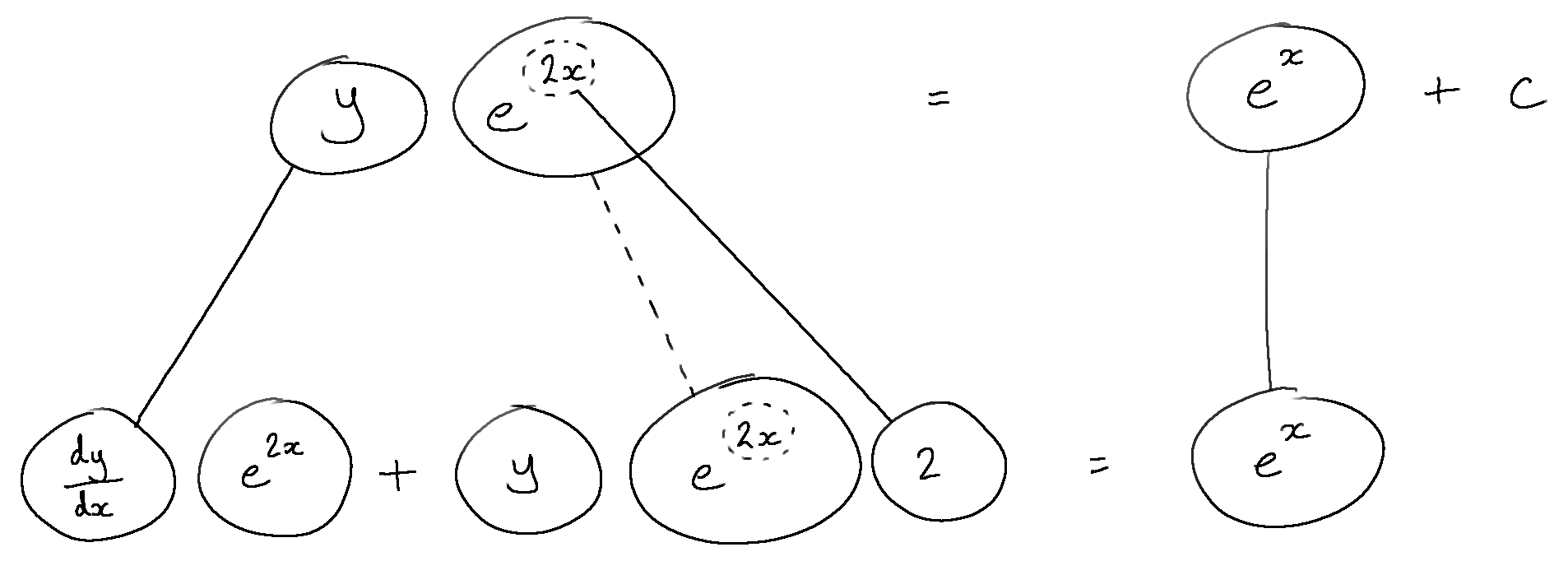# Thread: First Order Linear Differential Equation

1. ## First Order Linear Differential Equation

Solve the differential equation

a) e^x (dy/dx)=1-2(e^x)y

For this one i divided by e^x to get dy/dx= 1/e^x -2y
which equals dy/dx-2y= 1/e^x

I think you are supposed to find an integrating factor. then go on to simplify.

b) 1+x (dy/dx)+y= sqrt(x)

for this one dy/dx=sqrt (x)/(1+x) -y/ (1+x) which equals dy/dx+(y/(1+x))= sqrt (x)/(1+x)

Similar to above I am obviously having trouble determining the integrating factor.

c) t (dy/dt)+2y=t^3
I also couldnt figure out the operating factor other ones worked out other than these ones. dy/dt+ (2y/t)= t^2

d) x (dy/dx) +y= sin x (x>0)
so, dy/dx+(y/x)= sinx/x

integrating e^ (int P(x)dx=e^(int 1/x)= e^lnx=x

so d/dx (xy)=sinx integrating both sides gives xy= -cosx+c

i am not sure if i did any of this right. Any help on all or even one of the question will be appreciated. Exam is in 2 days and I really am trying to get this all in my head... p.s. For people who think i didn't try to solve this, believe me when i say i spent hours trying to figure these differential questions by reading notes and internet.

2. Just in case a picture helps...... where (key in spoiler) ...

Spoiler:... is the chain rule. Straight continuous lines differentiate downwards (integrate up) with respect to the main variable (in this case ), and the straight dashed line similarly but with respect to the dashed balloon expression (the inner function of the composite which is subject to the chain rule).

However, the chain rule is embedded in the legs-uncrossed version of...... the product rule. (Straight continuous lines still differentiating down and integrating up with respect to x.)

The general drift is...Then...Spoiler:_________________________________________

Don't integrate - balloontegrate!

Balloon Calculus; standard integrals, derivatives and methods

Balloon Calculus Drawing with LaTeX and Asymptote!

3.Originally Posted by dreamx20Solve the differential equation

a) e^x (dy/dx)=1-2(e^x)y

For this one i divided by e^x to get dy/dx= 1/e^x -2y
which equals dy/dx-2y= 1/e^x

I think you are supposed to find an integrating factor. then go on to simplify.
Yes, that is correct. And an integrating factor is a function $\displaystyle \mu(x)$ such that $\displaystyle \frac{d(\mu(x) y)}{dx}= \mu(x)\frac{dy}{dx}- 2\mu(x)y$. Differentiating on the left, using the product rule, gives $\displaystyle \mu(x)\frac{dy}{dx}+ \frac{d\mu}{dx}y= \mu(x)\frac{dy}{dx}- 2\mu(x)y$ so $\displaystyle \mu(x)$ must satisfy $\displaystyle \frac{d\mu}{dx}= -2\mu$.

b) 1+x (dy/dx)+y= sqrt(x)

for this one dy/dx=sqrt (x)/(1+x) -y/ (1+x) which equals dy/dx+(y/(1+x))= sqrt (x)/(1+x)

Similar to above I am obviously having trouble determining the integrating factor.
Again, you want $\displaystyle \mu(x)$ such that $\displaystyle \frac{d\mu}{dx}= \frac{\mu}{1+ x}$. Integrate $\displaystyle \frac{d\mu}{\mu}= \frac{dx}{1+ x}$.

c) t (dy/dt)+2y=t^3
I also couldnt figure out the operating factor other ones worked out other than these ones. dy/dt+ (2y/t)= t^2
So the integrating factor satisfies $\displaystyle \frac{d\mu}{dt}= \frac{2\mu}{t}$ or [tex]\frac{d\mu}{\mu}= \frac{2dt}{t}. That should be easy to integrate.

d) x (dy/dx) +y= sin x (x>0)
so, dy/dx+(y/x)= sinx/x
And the integrating factor satisfies $\displaystyle \frac{d\mu}{dx}= \frac{\mu}{x}$. $\displaystyle \frac{d\mu}{\mu}= \frac{dx}{x}$.

[quote]integrating e^ (int P(x)dx=e^(int 1/x)= e^lnx=x

so d/dx (xy)=sinx integrating both sides gives xy= -cosx+c[quote]
Yes that is correct.

i am not sure if i did any of this right. Any help on all or even one of the question will be appreciated. Exam is in 2 days and I really am trying to get this all in my head... p.s. For people who think i didn't try to solve this, believe me when i say i spent hours trying to figure these differential questions by reading notes and internet.
For people who think you didn't try to solve this, the best thing to do would be to show what you have tried. You don't solve problems like these "by reading notes and internet"- you solve problems like these by trying many different things.

4. However, I forgot to mention above that the OP has -2y in his or her transformed (first) equation but it wants to be +2y.

#### Search Tags

differential, equation, linear, order